# FFT详解

## 最新更新

2018.6.29 新增了“关于DFT的再次思考” 和 “有关FFT算法实现机理的再讨论”两个小节，希望能对没学明白的同学有所帮助。

2018.6.23 回复了评论区的两个评论，如果同学们觉得我的讲解有错误，或者是有讲得不够清晰的地方，请在评论区评论评论，我会虚心采纳的，谢谢。

2017.12.8 利用$LATEX$$LATEX$对公式进行重新整理，用以辅助原来的图片公式。但是为了体现出这篇博客的”历史沧桑感”，我保留了原有的所有图片（暂时只完成了一部分的公式转换，公式实在是太多了！）。—— GGN

## 序言

（来学习的同学们请直接跳过这里就好了…）

2017.11.19 GGN 补加序言

## 前言

Leo 的 《FFT与多项式乘法

（温馨提示：整篇文章都不是很好理解，提醒同学们保持清醒的头脑。）

## 1.什么是FFT？

FFT，即为快速傅氏变换，是离散傅氏变换的快速算法，它是根据离散傅氏变换的奇、偶、虚、实等特性，对离散傅立叶变换的算法进行改进获得的。它对傅氏变换的理论并没有新的发现，但是对于在计算机系统或者说数字系统中应用离散傅立叶变换，可以说是进了一大步。——360百科

FFT（Fast Fourier Transformation）就是“快速傅里叶变换”的意思，它是一种用来计算DFT（离散傅里叶变换）和IDFT（离散傅里叶反变换）的一种快速算法。这种算法运用了一种高深的数学方式、把原来复杂度为$O\left({n}^{2}\right)$$O(n^2)$的朴素多项式乘法转化为了$O\left(nlogn\right)$$O(nlog n)$的算法。

## 2.多项式乘法的朴素算法

$f\left(x\right)={a}_{1}{x}^{2}+{b}_{1}x+{c}_{1}$

$g\left(x\right)={a}_{2}{x}^{2}+{b}_{2}x+{c}_{2}$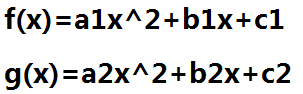$K\left(x\right)=f\left(x\right)×g\left(x\right)={a}_{1}{a}_{2}{x}^{4}+\left({a}_{1}{b}_{2}+{a}_{2}{b}_{1}\right){x}^{3}+\left({a}_{1}{c}_{2}+{a}_{2}{c}_{1}+{b}_{1}{b}_{2}\right){x}^{2}+\left({b}_{1}{c}_{2}+{b}_{2}{c}_{1}\right)x+{c}_{1}{c}_{2}$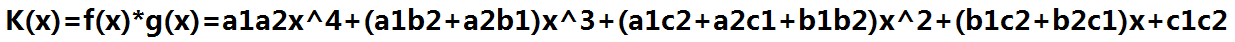#include<iostream>
#include<vector>
#include<cstdlib>
using namespace std;

vector<double>ForceMul(vector<double>A,vector<double>B)//表示A,B两个多项式相乘的结果
{
vector<double>ans;
int aLen=A.size();//A的元素个数
int bLen=B.size();//B的元素个数
int ansLen=aLen+bLen-1;//ans的元素个数=A的元素个数+B的元素个数-1
for(int i=1;i<=ansLen;i++)//初始化ans
ans.push_back(0);
for(int i=0;i<aLen;i++)
for(int j=0;j<bLen;j++)
ans[i+j]+=A[i]*B[j];//A的i次项 与 B的j次项 相乘的结果 累加到ans的[i+j]次位
return ans;//返回ans
}

int main()
{
vector<double>A,B;
cout<<"input A:";
for(int i=0;i<3;i++)//从0次项开始输入A的各项系数
{
int x;
cin>>x;
A.push_back(x);
}
cout<<"input B:";
for(int i=0;i<3;i++)//从0次项开始输入B的各项系数
{
int x;
cin>>x;
B.push_back(x);
}
vector<double>C=ForceMul(A,B);//C=A与B暴力相乘
cout<<"output C:";
for(int i=0;i<5;i++)//从0次项开始输出C的各项系数
cout<<C[i]<<" ";
cout<<endl;
system("pause");
return 0;
}


## 3.系数表示法与点值表示法

$f\left(x\right)={a}_{0}+{a}_{1}x+{a}_{2}{x}^{2}+..+{a}_{n}{x}^{n}⇔f\left(x\right)=\left\{{a}_{0},{a}_{1},{a}_{2},..,{a}_{n}\right\}$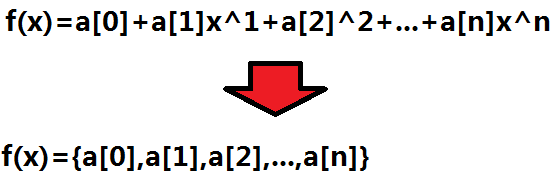（两点确定一条直线，三点确定一条抛物线…同理n+1个点确定一个n次函数，其原理来自于“高斯消元”，下文会有介绍。）

$f\left(x\right)={a}_{0}+{a}_{1}x+{a}_{2}{x}^{2}+..+{a}_{n}{x}^{n}⇔f\left(x\right)=\left\{\left({x}_{0},{y}_{0}\right),\left({x}_{1},{y}_{1}\right),\left({x}_{2},{y}_{2}\right),..,\left({x}_{n},{y}_{n}\right)\right\}$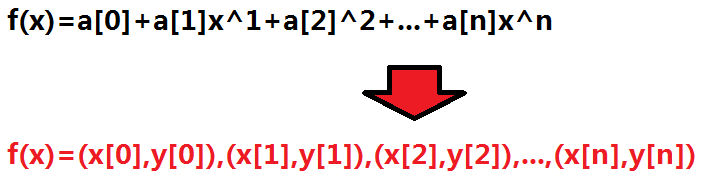$f\left({x}_{0}\right)={y}_{0}={a}_{0}+{a}_{1}{x}_{0}+{a}_{2}{x}_{0}^{2}+..+{a}_{n}{x}_{0}^{n}$

$f\left({x}_{1}\right)={y}_{1}={a}_{0}+{a}_{1}{x}_{1}+{a}_{2}{x}_{1}^{2}+..+{a}_{n}{x}_{1}^{n}$

$f\left({x}_{2}\right)={y}_{2}={a}_{0}+{a}_{1}{x}_{2}+{a}_{2}{x}_{2}^{2}+..+{a}_{n}{x}_{2}^{n}$

$...$

$f\left({x}_{n}\right)={y}_{n}={a}_{0}+{a}_{1}{x}_{n}+{a}_{2}{x}_{n}^{2}+..+{a}_{n}{x}_{n}^{n}$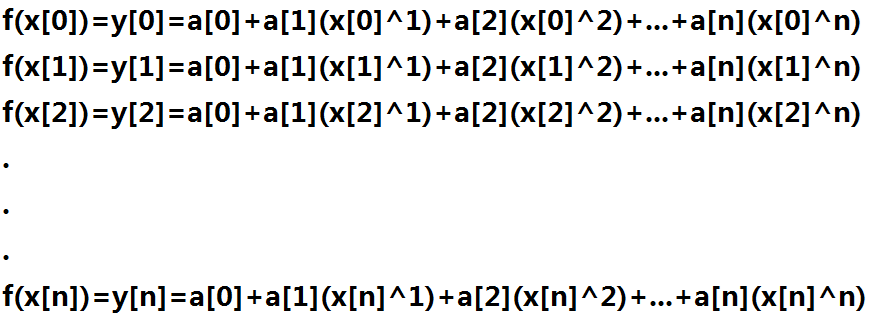（请同学们重视上面的这两句话，因为这是我能想到的最好理解的解释方法了。）

## 4.“复数”的引入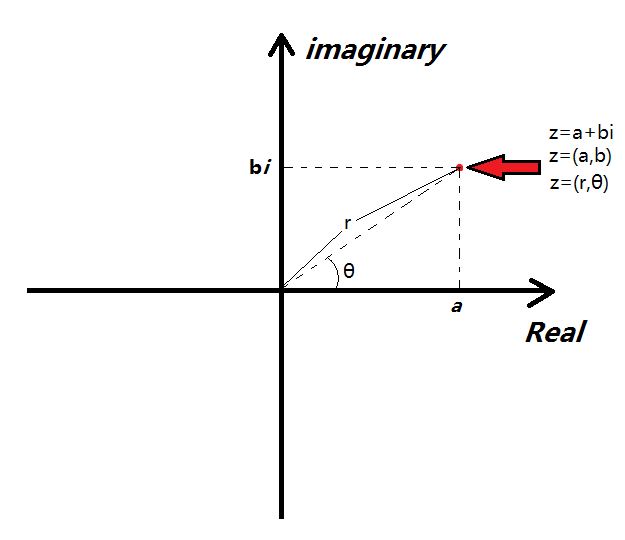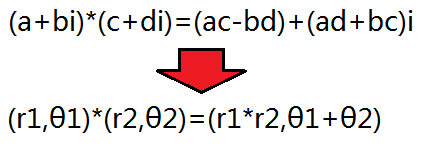## 5.单位复根

$f\left(x\right)=\left\{\left({x}_{0},f\left({x}_{0}\right)\right),\left({x}_{1},f\left({x}_{1}\right)\right),\left({x}_{2},f\left({x}_{2}\right)\right),..,\left({x}_{n},f\left({x}_{n}\right)\right)\right\}$

$g\left(x\right)=\left\{\left({x}_{0},g\left({x}_{0}\right)\right),\left({x}_{1},g\left({x}_{1}\right)\right),\left({x}_{2},g\left({x}_{2}\right)\right),..,\left({x}_{n},g\left({x}_{n}\right)\right)\right\}$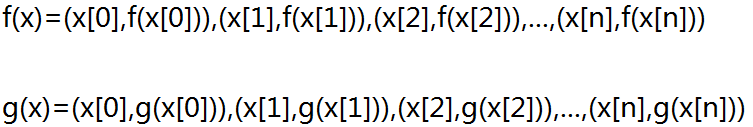$f\left(x\right)×g\left(x\right)=\left\{\left({x}_{0},f\left({x}_{0}\right)g\left({x}_{0}\right)\right),\left({x}_{1},f\left({x}_{1}\right)g\left({x}_{1}\right)\right),\left({x}_{0},f\left({x}_{2}\right)g\left({x}_{2}\right)\right),..,\left({x}_{n},f\left({x}_{n}\right)g\left({x}_{n}\right)\right)\right\}$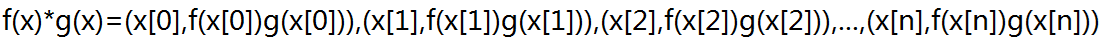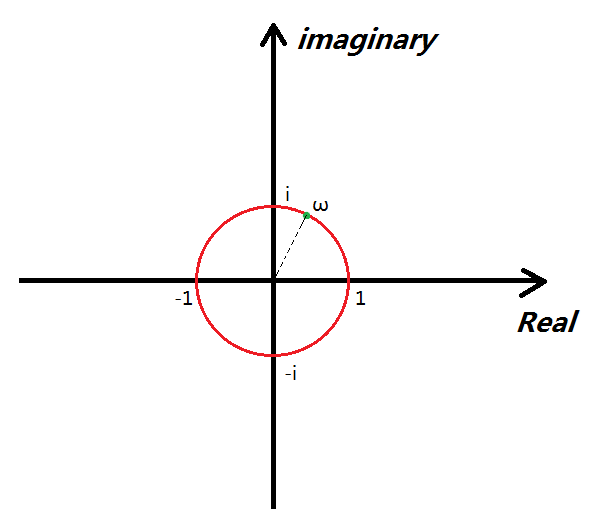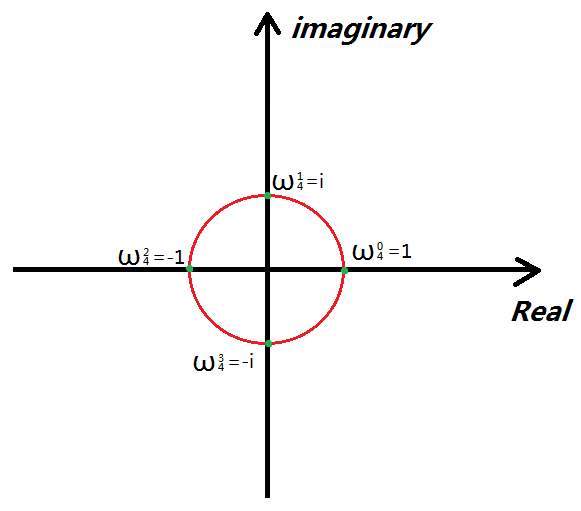## 6.FFT的主要流程之DFT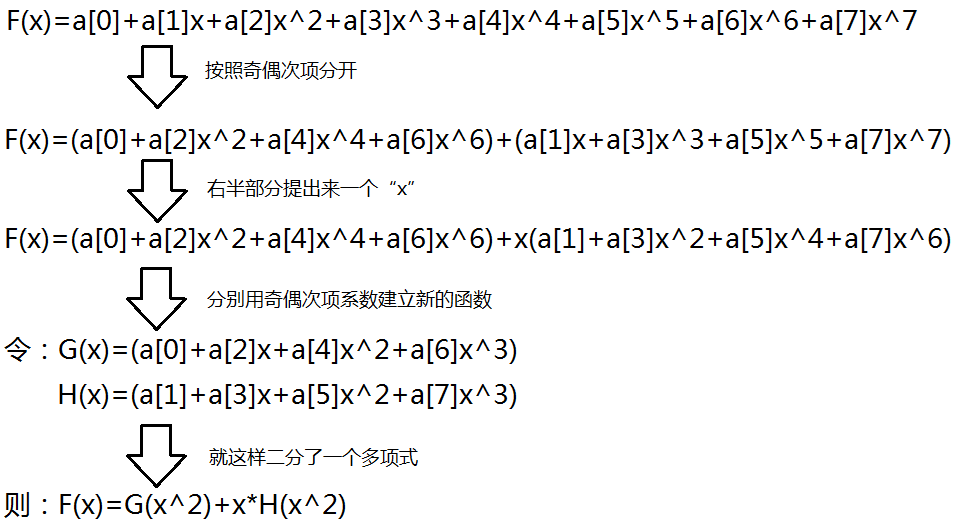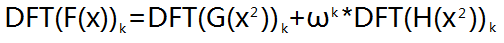（把当前单位复根的平方分别以DFT的方式带入G函数和H函数求值。）

【2018.6.30】 我觉得上面这一段内容讲得还不够清晰，如果同学们没明白这段话的意思，可以先跳到后面“关于DFT的再次思考”，看看那里的讲解。看完以后再回到这里，继续阅读。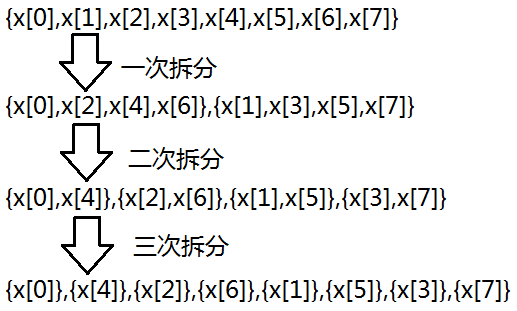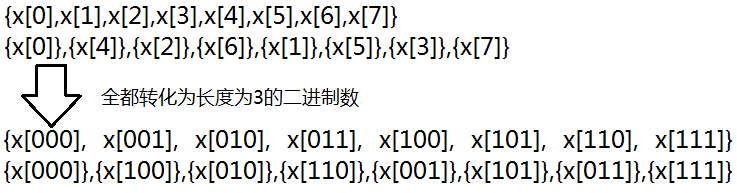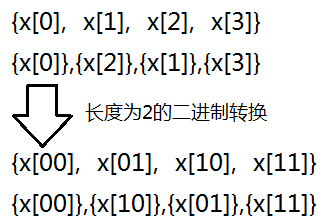（一个数学性质，在此不再证明。我感觉这个原理有点像是“基数排序”，感兴趣的同学可以去看看。）

## 7.FFT的主要流程之IDFT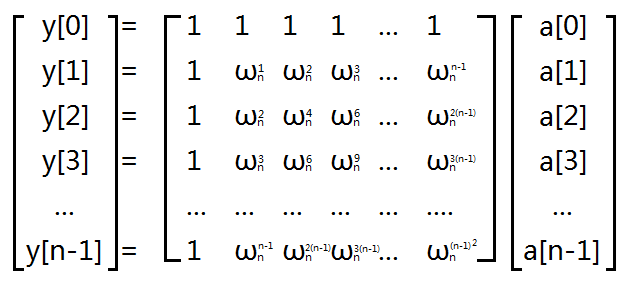int rev[maxl];
void get_rev(int bit)//bit表示二进制位数,计算一个数在二进制翻转之后形成的新数
{
for(int i=0;i<(1<<bit);i++)
rev[i]=(rev[i>>1]>>1)|((i&1)<<(bit-1));
}
void fft(cd *a,int n,int dft)//n表示我的多项式位数
{
for(int i=0;i<n;i++) if(i<rev[i]) swap(a[i],a[rev[i]]);
//中间的那个if保证了每个数做多只被交换了1次
//如果不写那么会有一些数被交换两次,导致最终的位置没有变
for(int step=1;step<n;step<<=1)//模拟一个合并的过程
{
cd wn=exp(cd(0,dft*PI/step));//计算当前单位复根
for(int j=0;j<n;j+=step<<1)
{
cd wnk(1,0);//计算当前单位复根
for(int k=j;k<j+step;k++)
{//蝴蝶操作
cd x=a[k];
cd y=wnk*a[k+step];
a[k]=x+y;//这就是上文中F(x)=G(x)+ωH(x)的体现
a[k+step]=x-y;
//后半个“step”中的ω一定和“前半个”中的成相反数
//“红圈”上的点转一整圈“转回来”，转半圈正好转成相反数
wnk*=wn;
}
}
}
if(dft==-1) for(int i=0;i<n;i++) a[i]/=n;
//考虑到如果是IDFT操作,整个矩阵中的内容还要乘上1/n
}

## 关于DFT的再次思考

2018.6.29 我最近有思考了一些关于DFT原理的问题，觉得之前讲得有些草率，决定再写一个表述更清晰一些的版本。每个人都有适合的自己讲课风格，如果没看懂上面的讲解，不妨试试看看这里。

1.我们通常用 ${\omega }_{n}={e}^{\frac{2\pi i}{n}}$$\omega_n = e^{\frac{2\pi i}{n}}$表示n次单位复根（的一次方）

2.n次单位复根的值随指数变化而循环，有 ${\omega }_{n}^{n+k}={\omega }_{n}^{k}$$\omega_n^{n+k}=\omega_n^k$，（也就是说k为整数时，${\omega }_{n}^{k}$$\omega_n^k$恰有n种不同的取值，分别为${\omega }_{n}^{0},{\omega }_{n}^{1},{\omega }_{n}^{2},...,{\omega }_{n}^{n-1}$$\omega_n^0,\omega_n^1,\omega_n^2,...,\omega_n^{n-1}$，k等于其余整数值时，都可以通过几次+n或者-n 平移 到这个区间里）

3.折半引理： ${\omega }_{n}^{k}={e}^{\frac{2\pi i}{n}\cdot k}={e}^{\frac{2\pi i}{\frac{n}{2}}\cdot \frac{k}{2}}={\omega }_{\frac{n}{2}}^{\frac{k}{2}}$$\omega_n^k = e^{\frac{2\pi i}{n} \cdot k} = e^{\frac{2\pi i}{\frac{n}{2}} \cdot \frac{k}{2}} = \omega_{\frac{n}{2}}^{\frac{k}{2}}$，这个性质一会儿会用到，一定要理解透彻。

A 为系数向量，${A}_{k}$$A_k$ 中储存原多项式中${x}^{k}$$x^k$项前的系数。
B 为点值向量，${B}_{k}$$B_k$ 中储存 $x={\omega }_{n}^{k}$$x = \omega_n^k$ 时原多项式的值。

${B}_{k}={A}_{0}+{A}_{1}\cdot \left({\omega }_{n}^{k}\right)+{A}_{2}\cdot \left({\omega }_{n}^{k}{\right)}^{2}+{A}_{3}\cdot \left({\omega }_{n}^{k}{\right)}^{3}+...+{A}_{n-1}\cdot \left({\omega }_{n}^{k}{\right)}^{n-1}$$B_k = A_0 + A_1 \cdot (\omega_n^k) + A_2 \cdot(\omega_n^k)^2 + A_3 \cdot(\omega_n^k)^3+...+A_{n-1}\cdot(\omega_n^k)^{n-1}$

${B}_{k}=\left({A}_{0}+{A}_{2}\cdot \left({\omega }_{n}^{k}{\right)}^{2}+{A}_{4}\cdot \left({\omega }_{n}^{k}{\right)}^{4}...+{A}_{n-2}\cdot \left({\omega }_{n}^{k}{\right)}^{n-2}\right)+\left({A}_{1}\cdot \left({\omega }_{n}^{k}\right)+{A}_{3}\cdot \left({\omega }_{n}^{k}{\right)}^{3}+{A}_{5}\cdot \left({\omega }_{n}^{k}{\right)}^{5}+...+{A}_{n-1}\cdot \left({\omega }_{n}^{k}{\right)}^{n-1}\right)$$B_k = (A_0+A_2\cdot(\omega_n^k)^2+ A_4 \cdot (\omega_n^k)^4...+A_{n-2}\cdot(\omega_n^k)^{n-2}) +(A_1\cdot(\omega_n^k)+A_3\cdot(\omega_n^k)^3+A_5\cdot(\omega_n^k)^5+...+A_{n-1}\cdot(\omega_n^k)^{n-1})$

${B}_{k}=\left({A}_{0}+{A}_{2}\cdot \left({\omega }_{n}^{k}{\right)}^{2}+{A}_{4}\cdot \left({\omega }_{n}^{k}{\right)}^{4}...+{A}_{n-2}\cdot \left({\omega }_{n}^{k}{\right)}^{n-2}\right)+{\omega }_{n}^{k}\cdot \left({A}_{1}+{A}_{3}\cdot \left({\omega }_{n}^{k}{\right)}^{2}+{A}_{5}\cdot \left({\omega }_{n}^{k}{\right)}^{4}+...+{A}_{n-1}\cdot \left({\omega }_{n}^{k}{\right)}^{n-2}\right)$$B_k = (A_0+A_2\cdot(\omega_n^k)^2+ A_4 \cdot (\omega_n^k)^4...+A_{n-2}\cdot(\omega_n^k)^{n-2}) +\omega_n^k\cdot(A_1+A_3\cdot(\omega_n^k)^2+A_5\cdot(\omega_n^k)^4+...+A_{n-1}\cdot(\omega_n^k)^{n-2})$

$\left({\omega }_{n}^{k}{\right)}^{2}={\omega }_{n}^{2k}={\omega }_{\frac{n}{2}}^{k}$$(\omega_n^k)^2=\omega_n^{2k} = \omega_{\frac{n}{2}}^{k}$

${B}_{k}=\left({A}_{0}+{A}_{2}\cdot \left({\omega }_{\frac{n}{2}}^{k}\right)+{A}_{4}\cdot \left({\omega }_{\frac{n}{2}}^{k}{\right)}^{2}...+{A}_{n-2}\cdot \left({\omega }_{\frac{n}{2}}^{k}{\right)}^{\frac{n}{2}-1}\right)+{\omega }_{n}^{k}\cdot \left({A}_{1}+{A}_{3}\cdot \left({\omega }_{\frac{n}{2}}^{k}{\right)}^{1}+{A}_{5}\cdot \left({\omega }_{\frac{n}{2}}^{k}{\right)}^{2}+...+{A}_{n-1}\cdot \left({\omega }_{\frac{n}{2}}^{k}{\right)}^{\frac{n}{2}-1}\right)$$B_k = (A_0+A_2\cdot( \omega_{\frac{n}{2}}^{k})+ A_4 \cdot ( \omega_{\frac{n}{2}}^{k})^2...+A_{n-2}\cdot( \omega_{\frac{n}{2}}^{k})^{\frac{n}{2}-1}) +\omega_n^k\cdot(A_1+A_3\cdot( \omega_{\frac{n}{2}}^{k})^1+A_5\cdot( \omega_{\frac{n}{2}}^{k})^2+...+A_{n-1}\cdot( \omega_{\frac{n}{2}}^{k})^{\frac{n}{2}-1})$

${B}_{k}={L}_{k}+{\omega }_{n}^{k}\cdot {R}_{k}$$B_k = L_k + \omega_n^k \cdot R_k$，其中$0\le k<\frac{n}{2}$$0\leq k < \frac{n}{2}$
${B}_{\frac{n}{2}+k}={L}_{k}-{\omega }_{n}^{k}\cdot {R}_{k}$$B_{\frac{n}{2}+k}=L_k - \omega_n^k \cdot R_k$，其中$0\le k<\frac{n}{2}$$0\leq k < \frac{n}{2}$

## 有关FFT算法实现机理的再讨论

$arr=\left\{{A}_{0},{A}_{1},...,{A}_{\frac{n}{2}-1},{A}_{\frac{n}{2}},{A}_{\frac{n}{2}+1},...,{A}_{n-1}\right\}$

$arr=\left\{{A}_{0},{A}_{2},...,{A}_{n-2},{A}_{1},{A}_{3},...,{A}_{n-1}\right\}$

$arr=\left\{{L}_{0},{L}_{1},...,{L}_{\frac{n}{2}-1},{R}_{0},{R}_{1},...,{R}_{\frac{n}{2}-1}\right\}$

$arr=\left\{{B}_{0},{B}_{1},...,{B}_{\frac{n}{2}-1},{B}_{\frac{n}{2}},{B}_{\frac{n}{2}+1},...,{B}_{n-1}\right\}$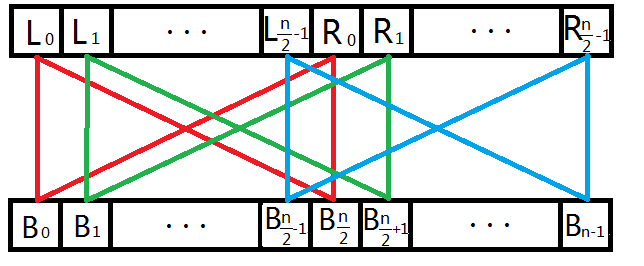$x={L}_{k},y={\omega }_{n}^{k}\cdot {R}_{k}$

${B}_{k}=x+y,{B}_{\frac{n}{2}+k}=x-y$

${\omega }_{n}^{k}={\omega }_{n}^{k-1}\cdot {\omega }_{n}$$\omega_n^k = \omega_n^{k-1} \cdot \omega_n$，是可以在循环的时候递推出来的。

IDFT的实现原理不再赘述，见上文第7节“FFT的主要流程之IDFT”。

int rev[maxl];
void get_rev(int bit)//bit表示二进制位数,计算一个数在二进制翻转之后形成的新数
{
for(int i=0;i<(1<<bit);i++)
rev[i]=(rev[i>>1]>>1)|((i&1)<<(bit-1));
}
void fft(cd *a,int n,int dft)//n表示我的多项式位数
{
for(int i=0;i<n;i++) if(i<rev[i]) swap(a[i],a[rev[i]]);
//中间的那个if保证了每个数做多只被交换了1次
//如果不写那么会有一些数被交换两次,导致最终的位置没有变
for(int step=1;step<n;step<<=1)//模拟一个合并的过程
{
cd wn=exp(cd(0,dft*PI/step));//计算当前单位复根
for(int j=0;j<n;j+=step<<1)
{
cd wnk(1,0);//计算当前单位复根
for(int k=j;k<j+step;k++)
{//蝴蝶操作
cd x=a[k];
cd y=wnk*a[k+step];
a[k]=x+y;//这就是上文中F(x)=G(x)+ωH(x)的体现
a[k+step]=x-y;
//后半个“step”中的ω一定和“前半个”中的成相反数
//“红圈”上的点转一整圈“转回来”，转半圈正好转成相反数
//一个数相反数的平方与这个数自身的平方相等..
wnk*=wn;
}
}
}
if(dft==-1) for(int i=0;i<n;i++) a[i]/=n;
//考虑到如果是IDFT操作,整个矩阵中的内容还要乘上1/n
}

## 8.后记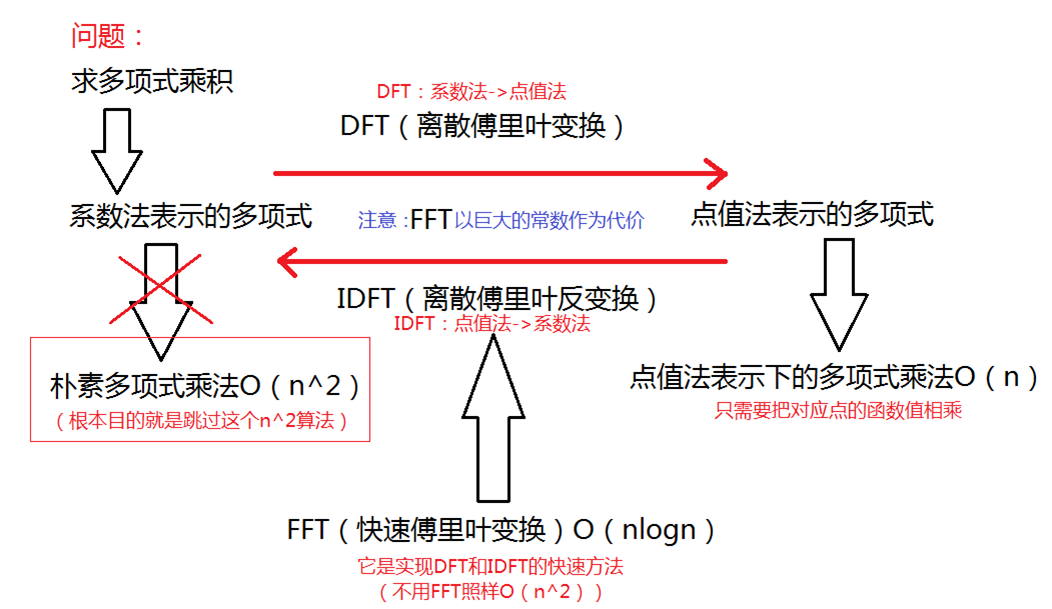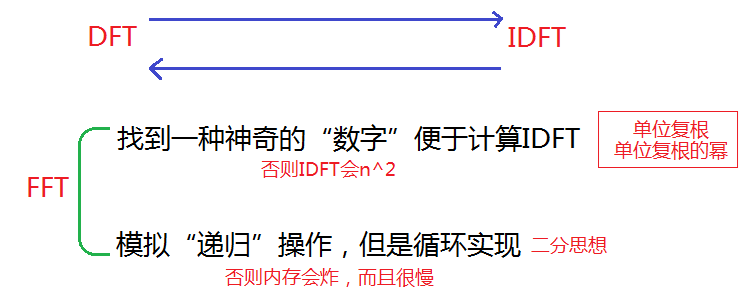（完全自创，如有雷同，纯属巧合，尽管并没什么用。）

FFT其实还可以用来计算BIGNUM乘法，因为我们可以把一个长整数理解成a+a*10+a*10^2+…+a[n]*10^n。把“10”当成未知数，这个多项式每一个次方项的系数就是BIGNUM每一数位上的数。而这时，数组长度“n”就不能单纯的取这个十进制数的长度，而要取大于等于两个十进制数长度加和的最小的2的正整数次幂。因为我们要保证DFT得到的离散点的个数足够表示我最终生成的新多项式（也就是取的点的个数要大于等于这个结果多项式的长度）。

2017.11.19【补】第一次自己手写FFT，感觉很开心

#include<cstdio>
#include<cstdlib>
#include<cmath>
#include<algorithm>
#include<cstring>
#include<complex>
using namespace std;

typedef complex<double> cd;//复数类的定义
const int maxl=2094153;//nlogn的最大长度(来自leo学长的博客)
const double PI=3.14159265358979;//圆周率,不解释

cd a[maxl],b[maxl];//用于储存变换的中间结果
int rev[maxl];//用于储存二进制反转的结果
void getrev(int bit){
for(int i=0;i<(1<<bit);i++){//高位决定二进制数的大小
rev[i]=(rev[i>>1]>>1)|((i&1)<<(bit-1));
}//能保证(x>>1)<x,满足递推性质
}

void fft(cd* a,int n,int dft){//变换主要过程
for(int i=0;i<n;i++){//按照二进制反转
if(i<rev[i])//保证只把前面的数和后面的数交换,(否则数组会被翻回来)
swap(a[i],a[rev[i]]);
}
for(int step=1;step<n;step<<=1){//枚举步长的一半
cd wn=exp(cd(0,dft*PI/step));//计算单位复根
for(int j=0;j<n;j+=step<<1){//对于每一块
cd wnk(1,0);//!!每一块都是一个独立序列,都是以零次方位为起始的
for(int k=j;k<j+step;k++){//蝴蝶操作处理这一块
cd x=a[k];
cd y=wnk*a[k+step];
a[k]=x+y;
a[k+step]=x-y;
wnk*=wn;//计算下一次的复根
}
}
}
if(dft==-1){//如果是反变换,则要将序列除以n
for(int i=0;i<n;i++)
a[i]/=n;
}
}

int output[maxl];
char s1[maxl],s2[maxl];
int main(){
scanf("%s%s",s1,s2);//读入两个数
int l1=strlen(s1),l2=strlen(s2);//就算"次数界"
int bit=1,s=2;//s表示分割之前整块的长度
for(bit=1;(1<<bit)<l1+l2-1;bit++){
s<<=1;//找到第一个二的整数次幂使得其可以容纳这两个数的乘积
}
for(int i=0;i<l1;i++){//第一个数装入a
a[i]=(double)(s1[l1-i-1]-'0');
}
for(int i=0;i<l2;i++){//第二个数装入b
b[i]=(double)(s2[l2-i-1]-'0');
}
getrev(bit);fft(a,s,1);fft(b,s,1);//dft
for(int i=0;i<s;i++)a[i]*=b[i];//对应相乘
fft(a,s,-1);//idft
for(int i=0;i<s;i++){//还原成十进制数
output[i]+=(int)(a[i].real()+0.5);//注意精度误差
output[i+1]+=output[i]/10;
output[i]%=10;
}
int i;
for(i=l1+l2;!output[i]&&i>=0;i--);//去掉前导零
if(i==-1)printf("0");//特判长度为0的情况
for(;i>=0;i--){//输出这个十进制数
printf("%d",output[i]);
}
putchar('\n');
return 0;
}

[2017.12.1]在此补充一下NTT（快速数论变换）的多项式乘法的代码。

#include<cstdio>
#include<cstdlib>
#include<algorithm>
#include<cmath>
//#include<complex>
using namespace std;
//typedef complex<double> cd;

typedef long long LL;

void exgcd(int a,int b,int& x,int& y){
if(b==0){
x=1;
y=0;
return;
}
int x0,y0;
exgcd(b,a%b,x0,y0);
x=y0;y=x0-int(a/b)*y0;
}

int Inv(int a,int p){
int x,y;
exgcd(a,p,x,y);
x%=p;
while(x<0)x+=p;
return x;
}

int qpow(int a,int b,int p){
if(b<0){
b=-b;
a=Inv(a,p);
}
LL ans=1,mul=a%p;
while(b){
if(b&1)ans=ans*mul%p;
mul=mul*mul%p;
b>>=1;
}
return ans;
}

#define maxn (65537*2)
const int MOD=479*(1<<21)+1,G=3;

int rev[maxn];
void get_rev(int bit){
for(int i=0;i<(1<<bit);i++){
rev[i]=(rev[i>>1]>>1)|((i&1)<<(bit-1));
}
}

//from internet
//for(int i=0; i<NUM; i++)
//{
//    int t = 1 << i;
//    wn[i] = quick_mod(G, (P - 1) / t, P);
//}

LL a[maxn],b[maxn];
void ntt(LL* a,int n,int dft){
for(int i=0;i<n;i++){
if(i<rev[i])
swap(a[i],a[rev[i]]);
}
for(int step=1;step<n;step<<=1){
LL wn;
wn=qpow(G,dft*(MOD-1)/(step*2),MOD);
for(int j=0;j<n;j+=step<<1){
LL wnk=1;//这里一定要用long long不然会迷之溢出
for(int k=j;k<j+step;k++){
LL x=a[k]%MOD,y=(wnk*a[k+step])%MOD;//这里也要用long long
a[k]=(x+y)%MOD;
a[k+step]=((x-y)%MOD+MOD)%MOD;
wnk=(wnk*wn)%MOD;
}
}
}
if(dft==-1){
int nI=Inv(n,MOD);
for(int i=0;i<n;i++)
a[i]=a[i]*nI%MOD;
}
}

#include<cstring>
char s1[maxn],s2[maxn];

int main(){
//scanf("%*d");
scanf("%s%s",s1,s2);
int l1=strlen(s1),l2=strlen(s2);
for(int i=0;i<l1;i++)a[i]=s1[l1-i-1]-'0';
for(int i=0;i<l2;i++)b[i]=s2[l2-i-1]-'0';
int bit,s=2;
for(bit=1;(1<<bit)<(l1+l2-1);bit++)s<<=1;
get_rev(bit);ntt(a,s,1);ntt(b,s,1);
for(int i=0;i<s;i++)
a[i]=a[i]*b[i]%MOD;
ntt(a,s,-1);
for(int i=0;i<s;i++){
a[i+1]+=a[i]/10;
a[i]%=10;
}
int cnt=s;
while(cnt>=0 && a[cnt]==0)cnt--;
if(cnt==-1)printf("0");
for(int i=cnt;i>=0;i--){
printf("%d",a[i]);
}
putchar('\n');
return 0;
}